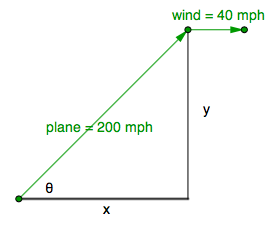### Home > PC3 > Chapter 10 > Lesson 10.2.2 > Problem10-125

10-125.

Write a set of parametric equations to model the position of a plane $t$ hours after takeoff if the plane is headed directly northeast at $200$ mph, and a wind is blowing east at $40$ mph.

Think vectors. What would the components be?

Draw and label a diagram.$\cos(\theta)=\frac{x}{200}$

Therefore $x = \:?$

The vectors in the diagram are velocity vectors.The problem asks for position. How do you calculate position given velocity?